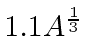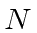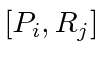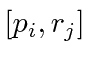## Homework 6

1. The energy spectrum of hydrogen can be written in terms of the principal quantum numberto be. What are the energies (in eV) of the photons from thetransition in hydrogen and deuterium? What is the difference in photon energy between the two isotopes of hydrogen?

2. Prove that the operator that exchanges two identical particles is Hermitian.

3. Two identical, non-interacting spinparticles are in a box. Write down the full lowest energy wave function for both particles with spin up and for one with spin up and the other spin down. Be sure your answer has the correct symmetry under the interchange of identical particles.

4. Ata particle is in the one dimensional Harmonic Oscillator state. Compute the expected value ofas a function of time.

5. Calculate the Fermi energy of a gas of massless fermions withparticles per unit volume.

6. The number density of conduction electrons in copper isper cubic centimeter. What is the Fermi energy in electron volts?

7. The volume of a nucleus is approximatelyFermis, where,is the number of neutrons, andis the number of protons. A Lead nucleus consists of 82 protons and 126 neutrons. Estimate the Fermi energy of the protons and neutrons separately.

8. The momentum operator conjugate to any cooridinateis. Calculate the commutators of the center of mass coordinates and momentaand of the internal coordinates and momenta. Calculate the commutatorsand.

Jim Branson 2013-04-22